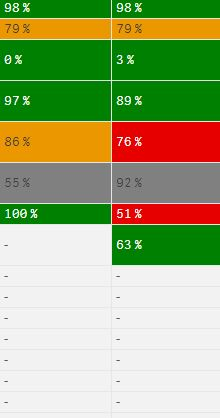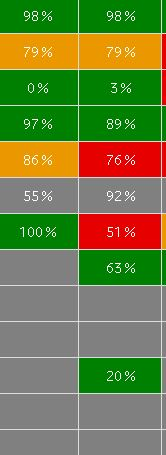# New to Qlik Sense

Discussion board where members can get started with Qlik Sense.

Highlighted
Contributor

## To eliminate Null Values in Pivot Table

Hi All,

I have a requirement to eliminate null values in Pivot table in Qliksense.

I have used the below expression as measure.

Only({\$<Year={'\$(=vlatestmnth)'},SC=,UT=,AC={'fieldfield'}>}SC &' ' &if(Unit='%','%'))

The Output as below.There are some null value displayed as '-'. Could please help how Can I remove these Null values and change the background colour to grey for these values.

My Output Should like as below.Note : I have unchecked the Include Null values in dimension Tab and Data Handling tab.

Thanks and Regards,

Kishore

9 Replies
Honored Contributor II

## Re: To eliminate Null Values in Pivot Table

if(isnull(Only({\$<Year={'\$(=vlatestmnth)'},SC=,UT=,AC={'fieldfield'}>}SC &' ' &if(Unit='%','%')))=-1,' ',

Only({\$<Year={'\$(=vlatestmnth)'},SC=,UT=,AC={'fieldfield'}>}SC &' ' &if(Unit='%','%')))

Learning never stops.
Contributor

## Re: To eliminate Null Values in Pivot Table

I am sorry the above expression is not working and still I could see the null Values '-''.

Thanks,

SK

Honored Contributor II

## Re: To eliminate Null Values in Pivot Table

you have two expression ? what are those ? can u post the app with less data or dummy data?

Learning never stops.MVP

## Re: To eliminate Null Values in Pivot Table

In the expression properties maybe set the text and background colour expressions to display grey if the expression evaluates to null().  The dash for null will still be there, but not be visible and both the text and the background will be the same grey.Partner

## Re: To eliminate Null Values in Pivot Table

a dump idea ! let''s see if it works;

change ur expresseion as follow:

if(Only({\$<Year={'\$(=vlatestmnth)'},SC=,UT=,AC={'fieldfield'}>}SC &' ' &if(Unit='%','%'))&'hello'='hello','',Only({\$<Year={'\$(=vlatestmnth)'},SC=,UT=,AC={'fieldfield'}>}SC &' ' &if(Unit='%','%')))

Contributor

## Re: To eliminate Null Values in Pivot Table

Hi Omar,

Its not working.

Honored Contributor II

## Re: To eliminate Null Values in Pivot Table

can you post sample for us to look into ?

Learning never stops.
Contributor

## Re: To eliminate Null Values in Pivot Table

I have used the below Background and Text Expressions but still I Could see the Null Values '-'.

Background Expression:
=if(Only({\$<Year={'\$(=vlatestmnth)'},SC=,UT=,RAG={'Green'},Code={'Year'}>}Year),\$(vGreen),
if(Only({\$<Year={'\$(=vlatestmnth)'},SC=,UT=,RAG={'Amber'},Code={'Year'}>}Year),\$(vAmber),
if(Only({\$<Year={'\$(=vlatestmnth)'},SC=,UT=,RAG={'Red'},Code={'Year'}>}Year),\$(vRed),RGB(128,128,128))))

Text Expression:
=if(Only({\$<Year={'\$(=vlatestmnth)'},SC=,UT=,RAG={'Green'},Code={'Year'}>}Year),White(),
if(Only({\$<Year={'\$(=vlatestmnth)'},SC=,UT=,RAG={'Amber'},Code={'Year'}>}Year),White(),
if(Only({\$<Year={'\$(=vlatestmnth)'},SC=,UT=,RAG={'Red'},Code={'Year'}>}Year),White(),RGB(255,255,255))))MVP

## Re: To eliminate Null Values in Pivot Table

Maybe try like this :

Background Expression:

=if(Only({\$<Year={'\$(=vlatestmnth)'},SC=,UT=,RAG={'Green'},Code={'Year'}>}Year),\$(vGreen),
if(Only({\$<Year={'\$(=vlatestmnth)'},SC=,UT=,RAG={'Amber'},Code={'Year'}>}Year),\$(vAmber),
if(Only({\$<Year={'\$(=vlatestmnth)'},SC=,UT=,RAG={'Red'},Code={'Year'}>}Year),\$(vRed),RGB(128,128,128))))

Text Expression:

=if(Only({\$<Year={'\$(=vlatestmnth)'},SC=,UT=,RAG={'Green'},Code={'Year'}>}Year),White(),
if(Only({\$<Year={'\$(=vlatestmnth)'},SC=,UT=,RAG={'Amber'},Code={'Year'}>}Year),White(),
if(Only({\$<Year={'\$(=vlatestmnth)'},SC=,UT=,RAG={'Red'},Code={'Year'}>}Year),White(),RGB(128,128,128))))

If still no joy, then you probably need to post a sample qvf.